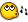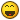## Recommended Posts

I only started yesterday so excuse the n00biness of the script

Anyway, this is supposed to follow a line but it's not working(My last problem was just that my pixelchecksum test wasn't working... because I mixed up the left and right co-ordinate...)

When I tested it... the mouse just went left

Anyway even if you can't help stay and chat cos I'm bored (and sad because my script failed)CODE
Global \$Paused

HotKeySet("{PAUSE}", "TogglePause")

HotKeySet("{ESC}", "Terminate")

Global \$Last = 0

Global \$Y

Global \$X

Global \$X = MouseGetPos(0)

Global \$Y = MouseGetPos(1)

Global \$left = \$X + \$Radius

Global \$top = \$Y + \$Radius

Global \$right = \$X - \$Radius

Global \$bottom = \$Y - \$Radius

Global \$color = PixelChecksum(\$left, \$top, \$right, \$bottom)

While 1

Global \$X = MouseGetPos(0)

Global \$Y = MouseGetPos(1)

if \$Last <> 1 Then

Up()

EndIf

if \$Last <> 2 Then

Right()

EndIf

If \$Last <> 3 Then

Down()

EndIf

If \$Last <> 4 Then

Left()

EndIf

WEnd

Func Up()

Local \$Vertical = 1

Local \$Horizontal = 0

Local \$Xleft = \$X - \$Radius

Local \$Ytop = \$Y +\$Vertical + \$Radius

Local \$Xright = \$X + \$Horizontal + \$Radius

Local \$Ybottom = \$Y - \$Radius

\$newcolor = PixelChecksum(\$Xleft, \$Ytop, \$Xright, \$Ybottom)

if \$newcolor >= \$color - 10 OR \$newcolor <= \$color + 10 Then

Local \$XChange = \$X + \$Horizontal

Local \$YChange = \$Y + \$Vertical

MouseMove(\$Xchange, \$Ychange, 1)

\$Last = 1

EndIf

EndFunc

Func Right()

Local \$Vertical = 0

Local \$Horizontal = 1

Local \$Xleft = \$X - \$Radius

Local \$Ytop = \$Y +\$Vertical + \$Radius

Local \$Xright = \$X + \$Horizontal + \$Radius

Local \$Ybottom = \$Y - \$Radius

\$newcolor = PixelChecksum(\$Xleft, \$Ytop, \$Xright, \$Ybottom)

if \$newcolor >= \$color - 10 OR \$newcolor <= \$color + 10 Then

Local \$XChange = \$X + \$Horizontal

Local \$YChange = \$Y + \$Vertical

MouseMove(\$Xchange, \$Ychange, 1)

\$Last = 2

EndIf

EndFunc

Func Down()

Local \$Vertical = -1

Local \$Horizontal = 0

Local \$Xleft = \$X - \$Radius

Local \$Ytop = \$Y +\$Vertical + \$Radius

Local \$Xright = \$X + \$Horizontal + \$Radius

Local \$Ybottom = \$Y - \$Radius

\$newcolor = PixelChecksum(\$Xleft, \$Ytop, \$Xright, \$Ybottom)

if \$newcolor >= \$color - 10 OR \$newcolor <= \$color + 10 Then

Local \$XChange = \$X + \$Horizontal

Local \$YChange = \$Y + \$Vertical

MouseMove(\$Xchange, \$Ychange, 1)

\$Last = 3

EndIf

EndFunc

Func Left()

Local \$Vertical = 0

Local \$Horizontal = -1

Local \$Xleft = \$X - \$Radius

Local \$Ytop = \$Y +\$Vertical + \$Radius

Local \$Xright = \$X + \$Horizontal + \$Radius

Local \$Ybottom = \$Y - \$Radius

\$newcolor = PixelChecksum(\$Xleft, \$Ytop, \$Xright, \$Ybottom)

if \$newcolor >= \$color - 10 OR \$newcolor <= \$color + 10 Then

Local \$XChange = \$X + \$Horizontal

Local \$YChange = \$Y + \$Vertical

MouseMove(\$Xchange, \$Ychange, 1)

\$Last = 4

EndIf

EndFunc

Func TogglePause()

\$Paused = NOT \$Paused

While \$Paused

sleep(1)

ToolTip('Script is "Paused"',0,0)

WEnd

ToolTip("")

EndFunc

Func Terminate()

Exit 0

EndFunc

##### Share on other sites

and adding to my complaints... I'm tired... its 12pm ...and I have school tomorrow... and I have a blocked nose...

*whines*

all that complaining made me feel betterEDIT: um... 12pm???!??! i wish... I meant 12am lol

Edited by alexx

##### Share on other sites

I just realised I could have shortened that script by making the horizontal and vertical variables in the loop... and had the single function... ah well... it still wouldn't work anyway so I'm not going to bother changing it now

##### Share on other sites

I just did shorten it anyway

CODE
Global \$Paused

HotKeySet("{PAUSE}", "TogglePause")

HotKeySet("{ESC}", "Terminate")

Global \$Last = 0

Global \$Y

Global \$X

Global \$X = MouseGetPos(0)

Global \$Y = MouseGetPos(1)

Global \$left = \$X + \$Radius

Global \$top = \$Y + \$Radius

Global \$right = \$X - \$Radius

Global \$bottom = \$Y - \$Radius

Global \$color = PixelChecksum(\$left, \$top, \$right, \$bottom)

Global \$Vertical = 0

Global \$Horizontal = 0

While 1

\$X = MouseGetPos(0)

\$Y = MouseGetPos(1)

if \$Last <> 1 Then

\$Vertical = 1

Test()

\$Vertical = 0

EndIf

if \$Last <> 2 Then

\$Horizontal = 1

Test()

\$Horizontal = 0

EndIf

If \$Last <> 3 Then

\$Vertical = -1

Test()

\$Vertical = 0

EndIf

If \$Last <> 4 Then

\$Horizontal = -1

Test()

\$Horizontal = 0

EndIf

WEnd

Func Test()

Local \$Xleft = \$X - \$Radius

Local \$Ytop = \$Y +\$Vertical + \$Radius

Local \$Xright = \$X + \$Horizontal + \$Radius

Local \$Ybottom = \$Y - \$Radius

\$newcolor = PixelChecksum(\$Xleft, \$Ytop, \$Xright, \$Ybottom)

if \$newcolor >= \$color - 10 OR \$newcolor <= \$color + 10 Then

Local \$XChange = \$X + \$Horizontal

Local \$YChange = \$Y + \$Vertical

MouseMove(\$Xchange, \$Ychange, 1)

\$Last = 1

if \$Vertical = 1 Then

\$Last = 1

Elseif \$Horizontal = 1 Then

\$Last = 2

Elseif \$Vertical = -1 Then

\$Last = 3

Elseif \$Horizontal = -1 Then

\$Last = 4

EndIf

EndIf

EndFunc

Func TogglePause()

\$Paused = NOT \$Paused

While \$Paused

sleep(1)

ToolTip('Script is "Paused"',0,0)

WEnd

ToolTip("")

EndFunc

Func Terminate()

Exit 0

EndFunc

##### Share on other sites

I just realised I could have shortened that script by making the horizontal and vertical variables in the loop... and had the single function... ah well... it still wouldn't work anyway so I'm not going to bother changing it now

Maybe explaining what you actually want your program to do in more detail than you already did would help. All anyone could do now is run your code and see what happens, but most probably no-one is "going to bother" to do that without some more information. Kindly inform the readers of this forum in detail what you expect your code to do, and where it goes wrong.

Even better would be to make a small test code which does nothing else than reproduce your problem, so other people know what to look for quicker. This will be much more inviting to troubleshoot.

Oh, and I hope your blocked nose gets better!Roses are FF0000, violets are 0000FF... All my base are belong to you.

##### Share on other sites

I didn't explain?

I'm trying to make a program that makes the mouse follow any line that is a certain colour and width (set by \$radius)

and the way i went about it was to imagine a circular object travelling on this line - so it tests the four directions for the colour and moves if its the same as the original colour... and so that it doesn't backtrack it doesn't go the same direction twice in a row

I can't think of any way to make it simpler## Create an account

Register a new account

• ### Recently Browsing   0 members

×

• Wiki

• Back

• #### Beta

• Git
• FAQ
• Our Picks
×
• Create New...